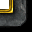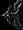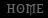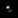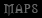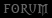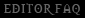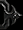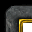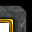# JASS: Deducting formulas

Tutorial By Daelin

1. Introduction
The biggest problem I&#8217;ve noticed that many JASS users have is the fact that they fail to improve their code (and thus, make it work faster), as they are not organized enough to get formulas rolling. I am not talking about super complicated ones, but in certain cases (such as working with coordinates), it is very important to reduce the number of calculations the processor must make. You may say that it is no noticeable, but spamming certain triggers (in big maps&#8230; but not necessarily) can cause extreme lag.

I will assume that you are already a decent JASS coder. The example based on which I will explain in this tutorial will be well known in the coding world, but it works with coordinates (so no more locations for you). That is because graphical patterns are usually the most formula-dependant. Some GUI users may learn certain things from this tutorial, but I wouldn&#8217;t put my bet on it (as code will be in JASS).
Okay then, let&#8217;s get started!

2. Important steps
Well, it is very important to identify the steps of the problem and keep it organized if you want to get a clean deduction and therefore, a correct and reliable formula. Don&#8217;t be afraid if you don&#8217;t know all mathematical concepts. There are a lot of websites (such as mathworld.com) which can help you greatly when you do not know certain important aspects. Yes, it may be difficult at the beginning, but in time, you will see that things can get so much easier. Google is your friend! So use it well, as in, use it every time you need something!

Identifying the problem
Now, the most important step is identifying the problem. For this tutorial, let&#8217;s say that you want to simulate a spell like Death Coil. Generally it is very simple, but the one problem we are facing is simulating the missile (no, it&#8217;s not efficient to rely on other spells to do the whole effect for you as you can&#8217;t control the spell that well). There are some tutorials out there that teach you how to move a unit from a point to another, but they clearly aren&#8217;t very efficient as they use a lot of trigonometric functions. They are time consuming! Those calculations could be spent somewhere else. Therefore, it would be so much better if we could use as few functions as possible. The calculations should be made every time we want to move the unit! This is extremely important, to think when you actually want to apply the &#8220;formula&#8221;.

Getting the input data
So we have the problem, let&#8217;s see what we actually &#8220;have&#8221;. The input data is very important, every time you get to such a problem, try to think clearly every element you could use to solve the problem. Two elements are already quite clear: the coordinates of the missile, respectively the target. Since we want to move the missile to the unit, we know these objects! Another thing we should know is the speed of the missile (how fast it moves). A last parameter is the frequency of the movement. You should know that this &#8220;moving&#8221; is done by repeatedly changing the position of the unit. How fast you change it, that&#8217;s the frequency (and it is obviously a constant, usually defined when starting the timer which does the trajectory).

Output data
Yes, we now have to see which output data we require. We want to move this unit repeatedly, very fast, and we only know a few stuff (where it is, when we want to go and how fast it can move). Therefore, we have to determine where the unit should find itself at the next step (it should come &#8220;forward&#8221; towards the target). If we can determine how much it should advance on the OX, respectively OY axis, we&#8217;ve solved the problem.

Actual calculations
Here comes the tricky part, as you have to use your input data (and possibly some other math formulas) to determine the next position of the unit. A simple alternative would be to use polar coordinates, but as I said before, they use trigonometric functions which are quite slow. Therefore, we need to manipulate input data in order to obtain output data. You will need a lot of practice to do this easily. Let&#8217;s see&#8230;

3. Solving the problem
It is not an uncommon fact that we need to work a lot with variables (hell&#8230; we usually work with them when deducting formulas even at math, physics, chemistry etc.). Therefore, let&#8217;s transfer the input data in &#8220;JASS&#8221; language. Sometimes it is important to make some notes on paper, usually with single letters and maybe indexes representing the input data. Here are my data:

v &#8211; missile speed
&#956; &#8211; missile frequency (timer speed)
d &#8211; initial distance
x1,y1 &#8211; missile coordinates
x2,y2 &#8211; target coordinates
---------------------------------------------
Of course, we also need intermediate values (obtained from initial values through some calculations), and for this problem, they are (we will see in a few moments how I got to them):

t &#8211; total time
tr &#8211; time left
&#916;x &#8211; distance between missile and target on OX axis
&#916;y &#8211; distance between missile and target on OY axis

Note: I&#8217;ve actually simplified the problem a little so that the missile always reaches its target in the same amount of time it would reach if the target did not move from the moment it is launched.

Usually we start the &#8220;thinking&#8221; by reducing the problem to an abstract level. For this problem, we want to determine the formula (where to move the missile next) regardless of the missile&#8217;s position, or that of the target. Here is a quick drawing (of course, the points could be anywhere):

[ATTACH]8003[/ATTACH]

To interpret it, I first need to explain you some elements. The triangles represent the missile (red), the target (blue), and the following position of the missile (cyan). &#916;x and &#916;y are quite obvious, as they are the distance between the two objects. And you can also clearly see that the coordinates of the next position are those of the initial, to which I added a value on the respective coordinate (on the X, respectively Y axis).

Ok, so we clearly know what we have to determine: those mysterious x+ and y+ stuff. Now, another thing you needed to figure out (yes, a bit difficult at first, I know, believe me, I do) is that you can actually determine how much time you have left until your missile much reach the target. How is that? Simple! Since we came to the conclusion (by simplifying the problem) that the time in which the missile must reach its target is constant, no matter how much it moves. So if the missile moves with 800 pixels per second and the initial distance between the caster and the target were 400 pixels, then the missile would reach its target in half a second (no matter how much the target moves). Therefore, we have out total time variable.

How we can detect the time left? Well, at each repetition of the timer (and therefore, each movement) we could reduce from the total time, how much it passed since the last movement (which is &#956;). And voila, we actually have the time left, at a certain moment. Now, let&#8217;s look again at the drawing. We know the distance, we know the total time, and we have to find a part of the distance for a certain moment. And here come proportions. Think about it like this: &#8220;if the target stood in place and the missile moved a repeated number of times, how many movements would it need to make in order to reach its target, knowing the total time and the frequency of the repetitions?&#8221;. I&#8217;d say it is that by dividing the time to the frequency, we actually get the number of movements.

Note: You need to initialize tr with the total time. To determine it, it&#8217;s enough to divide the initial distance between the caster and the target and divide it to the speed of the missile.

Example: Let&#8217;s say that you have to move a missile in two seconds, with a frequency of 0.05 seconds between the movements. Well, you would need two split those two seconds into small periods of two seconds. The number of periods would actually be the number of times you need to move your missile. In this example, that value would be 40.

I will name this variable Q (number of movements), and therefore, we have the following relation: Q=tr/ &#956;. Why I based it off the time left and not the initial time? Because we are interested in the number of repetitions required at a certain moment, not at the beginning. Generalization

And now we go back to the graphic. If the total distance depends on the time left, then so does the current distance modification (x+ respectively y+) depend on the frequency. I suspect many of you learned proportions at math, and so, know about a nice rule which would look like this (it is the same for &#916;y):

&#916;x.......................................... tr
x+.......................................... &#956;

From here, x+ = &#916;x * &#956; / tr
But &#956; / tr = 1/Q
Therefore x+ = &#916;x/Q (and in the same manner y+ = &#916;y/Q)

However, we still have some problems about &#916;x (and therefore similar to &#916;y). Consider different situations for the two points (when one is to the left or right of another). We should study it on cases and see how the formula behaves.

Case 1
x1<x2 => missile is in the left of the target => we need to increase the x coordinate => x+ > 0 => &#916;x>0 => |x1-x2|>0 (where |a| is the absolute value of a) => |x1-x2|=x2-x1
Therefore: &#916;x=x2-x1

Case 2
x1>x2 => missile is in the right of the target => we need to decrease the x coordinate => x+ < 0 => &#916;x<0 => |x1-x2|<0 => |x1-x2|=x2-x1
Therefore: &#916;x=x2-x1

Case 3
x1=x2 => &#916;x=x2-x1=x1-x2=0 (sign does not matter as absolute value is 0)

From these three we can conclude that &#916;x=x2-x1, no matter the position of the two points. Similarly, &#916;y=y2-y1.
Note: &#956; / tr is always greater than 0. Therefore the sign of x+ and y+ always depends on &#916;x or &#916;y.

And done, having finalized our problem, here is how our solution would look (brief, with no explanations):

t = &#8730;[(x2-x1)&#178;+(y2-y1)&#178;]/v
tr &#8211; initialized with t, reduced at each timer loop with &#956;
&#916;x = x2-x1
&#916;y = y2-y1
Q = tr/ &#956;
x+ = &#916;x/Q
y+ = &#916;y/Q

Exercise: Improve the formula so that you do not need to calculate Q every time we move the missile (at every repetition). Think that Q depends on &#956; (which is a constant), and tr, which also depends on &#956; and decreases every step.

Tip
You may sometimes have to rely on logical solutions, as you cannot always &#8220;catch&#8221; the idea mathematically. The proportions I presented when solving x+/y+ have a logical fundament in basis, and in this case, it is correct universally. Be careful though, that in some cases, you may not be able to fully rely on a logical hunch, and that&#8217;s why your formula may be wrong. It takes time and practice to get how these things work.

4. Implementing the formula
This is very simple. I shall spend though a few minutes for this problem too, as some of you might find it confusing. First thing to do is transform the data names into correct variable names.

&#956; = u
&#916;x = dx
&#916;y = dy
x+ = xp
y+ = yp

Tip
If you have been using indexes (I personally wrote on the paper tr with r as an index, same for x1,x2,y1,y2&#8230; for x+ and y+ I placed the &#8216;+&#8217; above the letter), just put them in front of the word. This way, you should be able to avoid any confusions of data (especially when there is a large number).

In the beginning, declare your constants into specific functions to avoid any problems later. The constants here are &#956; and v.
constant function Missile_Speed takes nothing returns real return 800.00 endfunction constant function Missile_Frequency takes nothing returns real return 0.035 endfunction

First of all, we create our first function which generates the missile and begins the movement. I split it into two functions to make it look good.

function Missile_BeginMovement takes unit missile, unit target returns nothing local real xx = GetUnitX(missile)-GetUnitX(target) //simplify distance function local real yy = GetUnitY(missile)-GetUnitY(target) // accordingly local real d = SquareRoot(xx*xx+yy*yy) //initial distance local real tr = d/Missile_Speed() //time left initialized with the total time local timer t = CreateTimer() //our timer call SetHandleHandle(t, "missile", missile) //I am using call SetHandleHandle(t, "target", target) //Kattana&#8217;s HandleVars cache call SetHandleHandle(t, "timeleft", tr) //you may use your own storing system call TimerStart(t, Missile_Frequency(), true, function Missile_StartTimer) call PolledWait(tr) //get out of the function only after the missile has reached its target set t = null endfunction function Missile_Main takes nothing returns nothing local unit cast = GetTriggerUnit() local unit targ = GetSpellTargetUnit() local unit missile = CreateUnit(GetOwningPlayer(cast), 'h000', GetUnitX(cast), GetUnitY(cast), 0.00) call Missile_BeginMovement(missile,target) //continue spell accordingly endfunction

Note: All input data are either linked to the timer or constants (which can be reached through functions). Other data can be easily determined using specific functions.

Okie dokie, things are quite clear. I used some helpful variables to determine the original distance so that I didn&#8217;t call coordinate determination functions eight times (only four). You may do so if you wish, as the efficiency problem is minimum, but I&#8217;m using to doing it how you&#8217;ve seen it above. Now let&#8217;s see that pesky timer function. I&#8217;ll explain after how I&#8217;ve actually implemented the formula (as this is the place where we do it!).

function Missile_Move takes timer t returns nothing local unit missile = GetHandleUnit(t, "missile") local unit target = GetHandleUnit(t, "target") local real tr = GetHandleReal(t, "timeleft") //INPUT DATA END local real dx = GetUnitX(target)-GetUnitX(missile) // &#916;x=x2-x1 local real dy = GetUnitY(target)-GetUnitY(missile) // &#916;y=y2-y1 local real xp = dx*Missile_Frequency()/tr local real yp = dy*Missile_Frequency()/tr call SetUnitX(missile, GetUnitX(missile)+xp) call SetUnitY(missile, GetUnitY(missile)+yp) set tr = tr- Missile_Frequency() //the reduction of time left I was telling you about if tr< Missile_Frequency() //in case Q is not an integer number call SetUnitX(missile, GetUnitX(target)) call SetUnitY(missile, GetUnitY(target)) call PauseTimer(t) call FlushHandleLocals(t) call DestroyTimer(t) else call SetHandleReal(t, "timeleft", tr) endif set missile = null set target = null endfunction function Missile_StartTimer takes nothing returns nothing call Missile_Move(GetExpiredTimer()) //let&#8217;s avoid any timer nulling problems endfunction

Note
That if/then/else stuff refers to the fact that if Q is not an integer number (there is not an integer number of segments), the number of repetitions would be screwed. Therefore, if we still have something like 0.032 left in tr, we have almost another repetition, but we can skip it as the difference is unnoticeable, and instantly move the unit to the target. If there is an even number of repetitions, once tr reaches 0, it is clear that its value is less than the frequency. Therefore, we destroy the timer and end the movement.

There, problem done! Test it and convince yourself that it works!

5. Mathematical concepts
Okay, this example was probably a bit simple, but I personally found it extremely relevant for the topic&#8230; took me a while to figure it out too, hehe. You will notice that math is really important if you want to optimize your code, because you can simplify your algorithm and/or expressions to make things easier. Here are some math elements that might help you.

Boolean expressions
Learn to simplify Boolean (logical) expressions, made out of 1 and 0 (which is translated into the language through true and false). The computer will strictly do what you tell him to do, so you will need to simplify them on paper. Here are some important relations which might help you (all variables are of type boolean):

[ATTACH]8002[/ATTACH]

Graph Theory
Though not with huge applicability in Warcraft at this point, as it hasn&#8217;t been exploited enough, graph theory is extremely useful for determining fast roads between units, roads of minimum cost (an optimal chain spell for example) and other things. Generally the nodes are units, and the edges are the path from one to another. Of course, because units actually move, the graphs are considered dynamic if calculations are made on a period of time and not in a certain moment. You can read more about graph theory on Wikipedia or here.

Analytical geometry
Spells work a lot with unit positions. In an abstract way, they are points in an XOYZ plane, as they have coordinates. Therefore many formulas may require that you have some solid knowledge into analytical geometry. Without studying this area you can&#8217;t really expect to optimize your code much (when it comes to positions and coordinates). You can read about analytical geometry on Wikipedia or Mathworld. Some interesting problems can also be studied here.

These are only a couple of areas you need to study. Of course, there are many more, but these are in my opinion the most important. Good luck and have fun developing and optimizing your JASS codes. And of course, if you have any comments or complaints about the tutorial, don&#8217;t hesitate to post them here. They will all be taken into consideration.